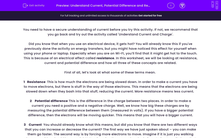# Understand Current, Potential Difference and Resistance

In this worksheet, students will revise the role of current, resistance and potential difference in circuits, as well as apply the relevant equation, V = IR.Key stage:  KS 4

Year:  GCSE

GCSE Boards:   AQA, AQA Trilogy, AQA Synergy, OCR 21st Century, OCR Gateway, Pearson Edexcel, Eduqas,

Popular topics:   Physics worksheets

Difficulty level:#### Worksheet Overview

You need to have a secure understanding of current before you try this activity. If not, we recommend that you go back and try out the activity called 'Understand Current and Charge'.

Did you know that when you use an electrical device, it gets hot? You will already know this if you've previously done the activity on energy transfers, but you might have noticed this effect for yourself when using your phone or laptop. Especially when you are on Wi-Fi, you’ll find that it might get hot to the touch. This is because of an electrical effect called resistance. In this worksheet, we will be looking at resistance, current and potential difference and how all three of these concepts are related.

First of all, let’s look at what some of these terms mean.

1   Resistance  This is how much the electrons are being slowed down. In order to make a current you have to move electrons, but there is stuff in the way of those electrons. This means that the electrons are being slowed down when they bash into that stuff, reducing the current. More resistance means less current.

2    Potential difference This is the difference in the charge between two places. In order to make a current you need a positive and a negative charge. Well, we know how big these charges are by measuring the potential difference between them (measured in volts). If you have a bigger potential difference, then the electrons will be moving quicker. This means that you will have a bigger current.

3    Current  You should already know what this means, but did you know that there are two different ways that you can increase or decrease the current? The first way we have just spoken about – you can make them go faster. The second way is by forcing more electrons to move. Imagine if it is just you walking down a corridor, should be easy right? Now imagine there are a hundred of you trying to walk down that same corridor – well, that’s a lot more difficult, isn’t it. This means there is more resistance if there is more current.

You should be able to see now how all three of these ideas are linked and we can see this in the most famous of all of the electrical equations:

V = IR

V = potential difference (measured in volts (V)) – also known as voltage.
I = current (measured in amperes or amps (A))
R = resistance (measured in ohms (Ω))

Now let’s take a look at that equation in action:

Question:  An electrical circuit has a current of 10 A and a total resistance of 3 Ω. Calculate the potential difference needed by this circuit.

Step 1   Highlight all of the numbers in the equation:

An electrical circuit has a current of 10 A and a total resistance of 3 Ω. Calculate the potential difference needed by this circuit.

Step 2   Write out the numbers next to their symbols:

V = ?
I = 10 A
R = 3 Ω

Step 3    Put the numbers into the equation:

V = 10 x 3

V = 30 V

Let’s try some questions on this!

### What is EdPlace?

We're your National Curriculum aligned online education content provider helping each child succeed in English, maths and science from year 1 to GCSE. With an EdPlace account you’ll be able to track and measure progress, helping each child achieve their best. We build confidence and attainment by personalising each child’s learning at a level that suits them.

Get started